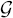## 14.5 THE INDEX-CALCULUS METHOD

Let q be a generator of a cyclic group= {1, 2, …, p − 1} of order p − 1 and y = qx (modulo p).

Proposition 14.5 (The Index-Calculus Algorithm): Initialization: Select a factor base= {p1, p2, …, ps} consisting of elements of.is chosen so that a significant proportion of the elements ofcan be expressed in the formwith ni ≥ 0.

 14.5a Select a random k with 0 ≤ k < n and compute qk (modulo p). 14.5b Try to write qk (modulo p) as a productwith ci, ≥ 0: if unsuccessful, return to Step 14.5a and choose another value for k; if successful, write k = [c1 logq p1 + [c2 logq p2 + … + [cs logq ps](modulo p − 1). 14.5c Repeat Steps 14.5a–b until a sufficient number of linear relations as above are found in order to solve the system of equations to determine ...

Get Computer Security and Cryptography now with the O’Reilly learning platform.

O’Reilly members experience live online training, plus books, videos, and digital content from nearly 200 publishers.